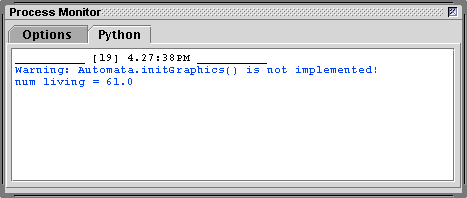### Basic Cellular Automata Python Classes

#### Introduction

This tutorial is part of a series that deal with the issues of programming cellular automata (CA) for use with Maya. This tutorial presents two python classes that implement the core functionality of a system that represents a 2D cellular automata. The classes will require subclassing in order to create graphics of the type described in Wikipedia's "Cellular Automaton".

Instances of the `Cell` class (listing 1) encapsulate the following data,
- the state of the cell ie. alive or dead,
- the previous state of the cell,
- the number of times the cell has been alive.
The initial state of a cell is decided by chance. Instances of the `Cell` class do not maintain any information about their own graphical representation or the state of their neighbors. It is left to subclasses of the `Cell` class to implement the code that creates a Maya shape that will represent the cell and its state.

An instance of the `Automata` class is responsible for,
- creating multiple instances of the `Cell` class, or instances of a subclass of `Cell`,
- maintaining a two dimensional list of cell instances ie. a list of lists of cells,
- applying Conways "rules of life" to determine the state of each cell,
Like the `Cell` class, the `Automata` class must be subclassed in order to create visually interesting results.

Listing 1 (AbstractAutomata.py)

 ```import random class Cell: def __init__(self, chance): self.prevState = 0 self.state = 0 self.lived = 0 if random.random() <= chance: self.prevState = 1 self.state = 1 self.lived += 1 def getState(self): return self.state ## Subclasses will override this method in order to ## update the appearance of the shape that represents ## a cell. def setState(self, state): self.state = state if state == 1: self.lived += 1 def getPrevState(self): return self.prevState def setPrevState(self, state): self.prevState = state def copyState(self): self.prevState = self.state def getNumLived(self): return self.lived #______________________________________________________________ class Automata: def __init__(self, numcols, numrows, chanceOfLife): self.rows = numrows self.cols = numcols self.numcells = numrows * numcols self.cells = [] # Make a (column) list. for i in range(numcols): column = [] # Make a list of (row) cells for each column for j in range(numrows): cell = self.getACell(chanceOfLife) column.append(cell) self.cells.append(column) self.initGraphics() ## Subclasses will override this method so that ## instances of their subclass of Cell will be used by ## the automata. def getACell(self, chanceOfLife): return Cell(chanceOfLife) ## Subclasses will override this method in order to ## position the shapes that represent the cells. def initGraphics(self): print("Warning: Automata.initGraphics() is not implemented!") def getAllCells(self): return self.cells def countLiving(self): numLiving = 0.0 for i in range(self.cols): for j in range(self.rows): numLiving += self.cells[i][j].getState() return numLiving def nextGeneration(self): # Move to the "next" generation for i in range(self.cols): for j in range(self.rows): self.cells[i][j].copyState() for i in range(self.cols): for j in range(self.rows): numLive = 0 iprev = i - 1 inext = i + 1 jprev = j - 1 jnext = j + 1 # When a cell is at the outer edge we "wrap" if i == 0: iprev = self.cols - 1 if j == 0: jprev = self.rows - 1 if i == self.cols - 1: inext = 0 if j == self.rows - 1: jnext = 0 # Query the state of the neighborhood. # Cells those above___ numLive += self.cells[iprev][jprev].getPrevState() numLive += self.cells[ i ][jprev].getPrevState() numLive += self.cells[inext][jprev].getPrevState() # Cells either side___ numLive += self.cells[iprev][ j ].getPrevState() numLive += self.cells[inext][ j ].getPrevState() # Cells below___ numLive += self.cells[iprev][jnext].getPrevState() numLive += self.cells[ i ][jnext].getPrevState() numLive += self.cells[inext][jnext].getPrevState() prevState = self.cells[i][j].getPrevState() currCell = self.cells[i][j] Automata.applyRulesOfLife(self, currCell, numLive) ## Subclasses can override this method in order to apply ## their own custom rules of life. def applyRulesOfLife(self, cell, liveNeighbors): if cell.prevState == 1: if liveNeighbors == 2 or liveNeighbors == 3: cell.setState(1) else: cell.setState(0) if cell.prevState == 0: if liveNeighbors == 3: cell.setState(1) else: cell.setState(0) ```

#### Testing the Classes

Save the contents of listing 1 in a file named AbstractAutomata.py. The file should be saved in a directory that will be sourced by Maya. For example, it could be saved in,

`    maya/scripts`

Alternatively, if the reader has followed the suggestions outlined in Maya: Setup for Python the file should be saved in,

`    maya/projects/RMS_python`

Listing 2 (TestAbstractClasses.py) provides the code for a script that will test the `Cell` and `Automata` classes. It should also be saved in the same directory as AbstractAutomata.py. Open the script in Cutter and run it by using the keyboard shortcut control + e, alt + e or apple + e. Cutter's Process Monitor should show text similar to that displayed in figure 1.

Listing 2 (TestAbstractClasses.py)

 ```import AbstractAutomata as abstract automata = abstract.Automata(10, 10, 0.5) for n in range(1): num = automata.countLiving() print("num living = %s" % num) automata.nextGeneration()```Figure 1

The next tutorial, "Algorithmic Form: CA PolyPlanes" demonstrates how `Cell` and `Automata` can be subclassed and used by Maya to display an animation of the "interaction" of the cells of a cellular automata.